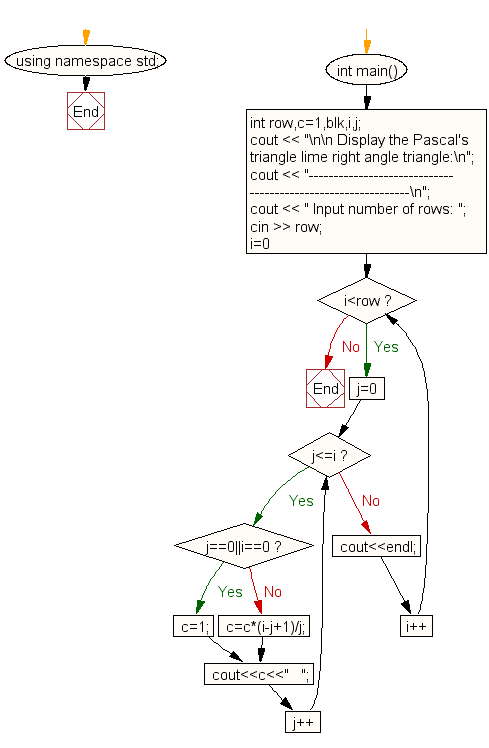﻿ C++ : Display Pascals triangle like right angle triangle# C++ Exercises: Display Pascal's triangle like right angle triangle

## C++ For Loop: Exercise-46 with Solution

Write a program in C++ to display Pascal's triangle like right angle triangle.

Sample Solution:-

C++ Code :

``````#include <iostream>
using namespace std;

int main()
{
int row,c=1,blk,i,j;
cout << "\n\n Display the Pascal's triangle lime right angle triangle:\n";
cout << "-------------------------------------------------------------\n";
cout << " Input number of rows: ";
cin >> row;
for(i=0;i<row;i++)
{
for(j=0;j<=i;j++)
{
if (j==0||i==0)
c=1;
else
c=c*(i-j+1)/j;
cout<<c<<"   ";
}
cout<<endl;
}
}
``````

Sample Output:

``` Display the Pascal's triangle lime right angle triangle:
-------------------------------------------------------------
Input number of rows: 7
1
1   1
1   2   1
1   3   3   1
1   4   6   4   1
1   5   10   10   5   1
1   6   15   20   15   6   1
```

Flowchart:C++ Code Editor:

Contribute your code and comments through Disqus.

What is the difficulty level of this exercise?

﻿

## C++ Programming: Tips of the Day

What is a smart pointer and when should I use one?

This answer is rather old, and so describes what was 'good' at the time, which was smart pointers provided by the Boost library. Since C++11, the standard library has provided sufficient smart pointers types, and so you should favour the use of std::unique_ptr, std::shared_ptr and std::weak_ptr.

There was also std::auto_ptr. It was very much like a scoped pointer, except that it also had the "special" dangerous ability to be copied - which also unexpectedly transfers ownership.

It was deprecated in C++11 and removed in C++17, so you shouldn't use it.

```std::auto_ptr<MyObject> p1 (new MyObject());
std::auto_ptr<MyObject> p2 = p1; // Copy and transfer ownership.
// p1 gets set to empty!
p2->DoSomething(); // Works.
p1->DoSomething(); // Oh oh. Hopefully raises some NULL pointer exception.
```

Ref : https://bit.ly/3mc9GHE